# 聚变堆液态金属包层MHD流动和传热数值模拟程序开发与验证Development and Validation of Numerical Simulation Program for MHD Flow and Heat Transfer in Liquid Metal Blanket of Fusion Reactor

DOI: 10.12677/NST.2019.73012, PDF, HTML, XML, 下载: 422  浏览: 808  国家自然科学基金支持

Abstract: The effect of magnetohydrodynamic effect on the flow and heat transfer of liquid metal in liquid blanket of fusion reactor is studied. A numerical simulation program of MHD flow and heat transfer distribution is developed. The flow and heat transfer phenomena in liquid blanket of fusion reactor are simulated by numerical method. The numerical simulation program is based on the four-step projection method to calculate the flow field distribution in the blanket under strong magnetic field. The temperature distribution is obtained by using the finite volume method. The results of numerical simulation are verified by standard examples and energy conservation methods. It provides a reference for the study of the flow and heat transfer characteristics of liquid metals in the blanket of fusion reactor.

1. 引言

2. 控制方程与数值方法

2.1. 控制方程

$\nabla \cdot u=0$ (1)

$\frac{\partial u}{\partial t}+u\cdot \nabla u=-\nabla p+\frac{1}{Re}\nabla \cdot \left(\nabla u\right)+N\left(J×B\right)$ (2)

${\nabla }^{2}\varphi =\nabla \cdot \left(u×B\right)$ (3)

$\frac{\partial T}{\partial t}+\nabla \cdot \left(uT\right)=\frac{1}{Pe}{\nabla }^{2}T+EcN{J}^{2}+\Phi$ (4)

p——压力；

B——磁感应强度；

J——电流密度；

T——温度。

2.2. 数值方法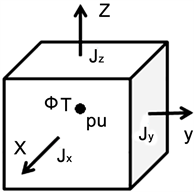Figure 1. Structural sketch of discrete grid

$\int \frac{\partial T}{\partial t}\text{d}v+\int \nabla \cdot \left(uT\right)\text{d}v=\left[\frac{1}{Pe}\int {\nabla }^{2}T\text{d}v+\int \left(EcN{J}^{2}+\Phi \right)\text{d}v\right]$ (5)

$\int \frac{\partial T}{\partial t}\text{d}v=\frac{T-{T}^{0}}{\Delta t}$ (6)

$\begin{array}{l}\int {\nabla }^{2}T\text{d}v=\left(\frac{{T}_{i+1,j,k}-{T}_{i,j,k}}{{x}_{i+1,j,k}-{x}_{i,j,k}}-\frac{{T}_{i,j,k}-{T}_{i-1,j,k}}{{x}_{i,j,k}-{x}_{i-1,j,k}}\right)\Delta y\Delta z+\left(\frac{{T}_{i,j+1,k}-{T}_{i,j,k}}{{y}_{i,j+1,k}-{y}_{i,j,k}}-\frac{{T}_{i,j,k}-{T}_{i,j-1,k}}{{y}_{i,j,k}-{y}_{i,j-1,k}}\right)\Delta x\Delta z\\ \text{\hspace{0.17em}}\text{\hspace{0.17em}}\text{\hspace{0.17em}}\text{\hspace{0.17em}}\text{\hspace{0.17em}}\text{\hspace{0.17em}}\text{\hspace{0.17em}}\text{\hspace{0.17em}}\text{\hspace{0.17em}}\text{\hspace{0.17em}}\text{\hspace{0.17em}}\text{\hspace{0.17em}}\text{\hspace{0.17em}}\text{\hspace{0.17em}}+\left(\frac{{T}_{i,j,k+1}-{T}_{i,j,k}}{{z}_{i,j,k+1}-{z}_{i,j,k}}-\frac{{T}_{i,j,k}-{T}_{i,j,k-1}}{{z}_{i,j,k}-{z}_{i,j,k-1}}\right)\Delta x\Delta y\end{array}$ (7)

$\int \nabla \cdot \left(uT\right)\text{d}v=\left(u{f}_{i+1,j,k}{T}_{i,j,k}-u{f}_{i-1,j,k}{T}_{i-1,j,k}\right)\Delta y\Delta z$ (8)

$\int \left(EcN{J}^{2}+\Phi \right)\text{d}v=\left(EcN{J}^{2}+\Phi \right)\Delta x\Delta y\Delta z$ (9)

2.3. 边界条件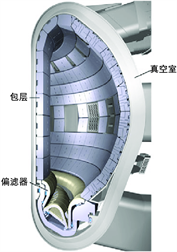Figure 2. Internal structure of ITER vacuum vessel

$\begin{array}{l}\frac{\text{d}T}{\text{d}x}=0\\ \frac{\text{d}T}{\text{d}y}=0\\ \frac{\text{d}T}{\text{d}z}=0\end{array}$ (10)

3. 结果与讨论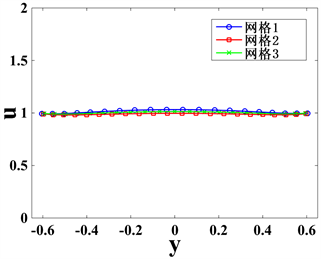Figure 3. Grid independence verification result

3.1. 速度分布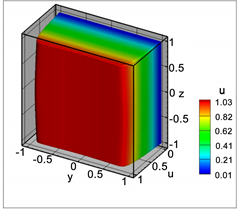(a)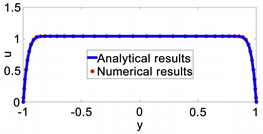(b)

Figure 4. Shercliff’s case results (Ha = 500, Re = 10); (a) Three dimensional velocity distribution of a cross section at x = 3.0; (b) Compare between numerical results and analytical results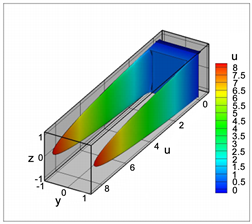(a)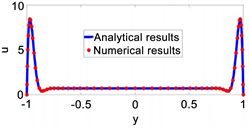(b)

Figure 5. Hunt’s case results (Ha = 1000, Re = 500); (a) Three dimensional velocity distribution of a cross section at x = 3.0; (b) Compare between numerical results and analytical resultsTable 1. Pressure gradient and flow rate for Shercliff’s case and Hunt’s case

3.2. 温度分布

$\Phi \cdot V=\sum \rho {c}_{p}u\left({T}_{out}-{T}_{in}\right)\Delta y\Delta z$ (11)

$V$ ——流道体积；

$\rho$ ——密度；

cp——比热容；

Tin——入口温度；

Tout——出口温度。

$\Delta \epsilon =\frac{|\Phi \cdot V-\sum \rho {c}_{p}u\left({T}_{out}-{T}_{in}\right)\Delta y\Delta z|}{\Phi \cdot V}$ (12)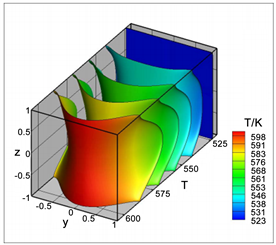Figure 6. Temperature distribution of Hunt case: Ha = 1000, Re = 500

4. 结论

NOTES

*通讯作者。

  Abdou, M.A., Morley, N.B. and Ying, A.Y. (2005) Overview of Fusion Blanket R&D in the US over the Last Decade. Nuclear Engineering and Technology, 37, 401-422.  Wong, C.P.C., Salavy, J.F., Kim, Y., Kirillov, I., Rajendra Kumar, E., Morley, N.B., et al. (2008) Overview of Liquid Metal TBM Concepts and Programs. Fusion Engineering and Design, 83, 850-857. https://doi.org/10.1016/j.fusengdes.2008.06.040  Shercliff, J.A. (1953) Steady Motion of Conducting Fluids in Pipes under Transverse Magnetic Fields. Proceedings of the Cambridge Philosophical Society, 49, 136-144. https://doi.org/10.1017/S0305004100028139  Hunt, J.C.R. (1965) Magnetohydrodynamic Flow in Rectangular Ducts. Journal of Fluid Mechanics, 21, 577-590. https://doi.org/10.1017/S0022112065000344  Ni, M.-J., Munipalli, R., Morley, N.B., Huang, P. and Abdou, M.A. (2007) A Current Density Conservative Scheme for Incompressible MHD Flows at a Low Magnetic Reynolds Number. Part I: On a Rectangular Collocated Grid System. Journal of Computational Physics, 227, 174-204. https://doi.org/10.1016/j.jcp.2007.07.025  Smolentsev, S., Morley, N.B., et al. (2008) MHD and Heat Transfer Considerations for the US DCLL Blanket for DEMO and ITER TBM. Fusion Engineering and Design, 83, 1788-1791. https://doi.org/10.1016/j.fusengdes.2008.04.002  袁保山, 姜韶风, 陆志鸿. 托卡马克装置工程基础[M]. 北京: 原子能出版社, 2011: 381-387.